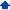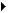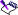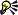### Recalculating formulas

You can set Quattro Pro to update calculations at specified intervals. By default, formulas are calculated when you enter them and recalculated each time you change the data. If a notebook contains many complex formulas, you may want to delay recalculation to save time.

You can set the order in which formulas recalculate depending on the nature of the formula. Available options are Natural, Column-wise, and Row-wise. You can also set the number of recalculation iterations.

#### To specify a calculation interval1 Click FormatNotebook properties.
 2 Click the Recalc settings tab.
 3 Enable one of the following options:
 • Automatic — recalculates formulas automatically, but pauses until recalculation is finished. Use Automatic if you have complex formulas that Background mode does not handle fast enough.
 • Manual — recalculates the formulas you enter or edit, but does not recalculate the entire notebook until you press F9. This setting is useful for large notebooks with many calculations.
 • Background — recalculates formulas between keystrokes. Background mode does not interrupt your work and recalculates automatically. Quattro Pro always finishes recalculating before saving, extracting, or printing the notebook.• The recommended recalculation setting is either Background or Automatic; both ensure that your data is accurate.• If you choose to use Manual mode, you can also recalculate single cells by selecting the cell, pressing F2, then pressing Enter.
 • You can manually calculate all formulas by clicking the calculator in the application bar; the calculator is enabled only when a formula needs to be recalculated.

#### To set the recalculation order1 Click FormatNotebook properties.
 2 Click the Recalc settings tab.
 3 Enable one of the following options:
 • Natural — calculates cells in dependency order. Formulas without dependencies are calculated first, followed by formulas that depend on them.
 • Column-wise — starts recalculation in cell A1 of the first sheet and proceeds down column A, ignoring formulas in other columns. When column A is finished, recalculation continues in cell B1, and down column B. This continues to the end of the sheet (column ZZZ), and on to the last sheet in the notebook.
 • Row-wise — starts recalculation in cell A1, but proceeds across the rows, starting at row 1 and continuing through to row 1,000,000, to the last sheet in the notebook.• If you enable the column-wise or row-wise options, you should set the number of iterations to at least two. Otherwise, the notebook may be inaccurate.

#### To set the number of recalculation iterations1 Click FormatNotebook properties.
 2 Click the Recalc settings tab.
 3 Type a value in the # of iterations box.• If the notebook has a formula with a circular cell reference, the formula’s cell address displays on the Recalc settings tab to the right of the # of iterations box. Elaborate formulas are sometimes deliberately constructed to contain circular cell references, because the formulas require multiple evaluations to attain an acceptable degree of accuracy.
 • If the notebook contains no circular cell references and the Natural option is enabled, the iteration count is ignored. For information on setting the recalculation order, see “To set the recalculation order.”